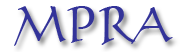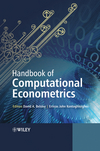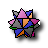Home

Concepts and tools for nonlinear time series modelling

Alessandra Amendola and Christian Francq
Keywords: Consistency and asymptotic normality; MCMC algorithms; Mixing; Nonlinear modelling; Stationarity; Time-series forecasting.
Abstract: A Tools and approaches are provided for nonlinear time series modelling in econometrics. A wide range of topics is covered, including probabilistic properties, statistical inference and computational methods. The focus is on the applications but the ideas of the mathematical arguments are also provided. Techniques and concepts are illustrated by various examples, Monte Carlo experiments and a real application.MPRA working paperHandbook of Computational Econometrics Edts: D. Belsley and E. Kontoghiorghes. Wiley 2009.
R and Mathematica code for the illustrations of the MPRA working paper :for the news impact curces of Figure 1 ,for the logistic functions of Figure 2 ,for the all-pass example (Example 3) of Figure 3,for the linearity tests of Figure 6 ,for the linearity tests of Table 2 ,for the stationarity regions of Figure 7 ,+for the stationarity regions of Figure 8 ,for the LSE of the EXPAR models of Figure 9 ,for the STAR models of Figure 11 ,for the metropolis algorithm on STAR models of Figure 14 ,for the hybrid Metropolis-Gibbs algorithm of Figure 15 ,for the Markov-Switching model on the CAC of Figure 16 .